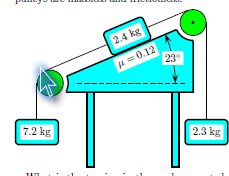# Unbalanced Forces on Ramp with Tension

## Homework Statement

The suspended 2.3 kg mass on the right is moving up, the 2.4 kg mass slides down the ramp, and the suspended 7.2 kg mass on the left is moving down. There is friction between the block and the ramp. The acceleration of gravity is 9.8 m/s2. The pulleys are massless and frictionless.

What is the tension in the cord connected to the 7.2 kg block? Answer in units of N.

Here is a photo:## Homework Equations

F=ma
Fg=mg
$$\mu$$=Ff/FN

## The Attempt at a Solution

I split it up into gravity parallel and gravity perpendicular and did the following to get those values:

sin23 * 23.52 = 9.19 N (parallel)
cos23 * 23.52 = 21.65 N (perpendicular)

Since the perpendicular force is equal to the normal force, the value of the normal force is also 21.65 N. Then I plugged the following to find the friction force:

$$\mu$$=Ff/FN

0.12 = Ff/21.65
Ff = 2.598 N

Then, I found the gravitational forces on the blocks hanging on the two sides.

7.2 kg * 9.8 m/s^2 = 70.56 N
2.3 kg * 9.8 m/s^2 = 22.54 N

This is the part I'm currently stuck on. Do I add up all the forces on the 2.4 kg block? Or do I have to use a summation to find the tension in the 7.2kg string?

The easiest way to do this problem is to find the equation of motion for the mass on the ramp. Call that mass m'.

Do vector addition for the forces on m'. The force from the ropes are in opposite directions, so they can be added easily. Call the heavier one m1 and the lighter m2. You can then find the acceleration of mass along the ramp.
$$a_{m'}=\frac{1}{m'}((m1-m2)g\sin \theta -\mu m'g\cos\theta)$$

Where theta is 23 degrees.
The term with the mu is the frictional force. Remember that for calculating the total tension in the left rope.

Now you have the acceleration of m', which is equal in magnitude to the accelerations of all the boxes. Remember that the downward force on the hanging boxes is not mg because they are accellerating: Fdown = m(g-a)

Note:I just edited that equation. By accident I left the right hand as the force--now it's devided my m' to be the correct acceleration.

Last edited: